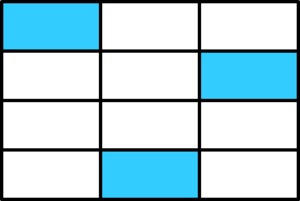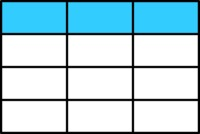# Fractions and Rectangles

Alignments to Content Standards: 4.NF.A.1

1. What fraction of the rectangle below is shaded?2. Laura says that $\frac14$ of the rectangle is shaded. Do you think she is correct? Explain why or why not by using the picture.

## IM Commentary

The primary goal of this task is for students to use pictures to explain the equivalence between $\frac{3}{12}$ and $\frac{1}{4}$. This is a step toward students understanding the general idea that

$$\frac{a\times n}{b\times n} = \frac{a}{b}$$

Students usually first see fraction equivalence by subdividing the pieces into smaller, equal sized pieces and realizing that you haven't changed the amount that represents the fraction. This explains why if you multiply the numerator and denominator by the same whole number, you get an equivalent fraction:

$$\frac{a}{b}=\frac{a\times n}{b\times n}$$

This task helps set students on the path to understanding that if you divide the numerator and denominator by the same whole number, you get an equivalent fraction. It is as if we are "putting smaller pieces together to form bigger pieces," as opposed to subdividing the pieces as seen in, e.g. 4.NF Explaining Fraction Equivalence with Pictures.

Students don't necessarily generate representations like the one shown in the task stem where the pieces are "scattered around," so it is good for them to see and think about such representations.

## Attached Resources

• Lesson Plan - Comparing Fractions 2
• ## Solution

1. In this picture the entire rectangle has been divided into 12 equal-sized pieces. So we can say that each piece is $\frac{1}{12}$ of the entire rectangle. Since 3 out of the total 12 pieces have been shaded, the shaded portion represents

$$\frac{1}{12}+\frac{1}{12}+\frac{1}{12} =3\times \frac{1}{12} = \frac{3}{12}$$

of the total rectangle. We can see the number in the denominator of the fraction represents how many pieces the entire rectangle is broken up into and the numerator represents how many of those pieces are shaded.

2. Laura is correct. We can see this by simply re-arranging the shaded parts of the rectangle to form a single row (See picture below.)To make it clear that this row represents $\frac14$ of the rectangle we can merge all of the pieces in a row together.Since the rectangle is now made up of 4 equal sized pieces, the shaded region (which is just one of the four pieces) represents $\frac14$ of the entire rectangle.